Contents

# Contents

## Idea

$6j$ symbols are pieces of combinatorial data which can be associated to fusion categories, encoding their associator with a finite amount of algebraic data. The $6j$ symbols along with the fusion ring is enough data to uniquely reconstruct the category.

$6j$ symbols are only defined for multiplicity free fusion categories. That is, fusion categories in which all of the fusion coefficients are $0$ or $1$. For fusion categories with larger coefficients, one has to replace $6j$ symbols (which are complex numbers) with higher-dimensional matrices.

The name “$6j$ symbol” comes from the fact that the symbol has $6$ indicies associated to it.

The $6j$ symbols depend on a local choice of basis of hom-spaces of your fusion category, and hence they are not bona-fide invariants. Choosing a different choice of local basis corresponds to a gauge transformation.

## Definition

Let $\mathcal{C}$ be a multiplicity free fusion category. Let

$\alpha: (- \otimes -)\otimes - \to - \otimes (-\otimes -)$

be its associator. We wish to encode the associator with a finite amount of algebraic data. That is, with a finite quantity of complex numbers. We do this by studying the action of $\alpha$ with respect to the (finite) set $\mathcal{L}$ of isomorphism classes of simple objects of $\mathcal{C}$.

For every triple $A,B,C$ of simple objects of $\mathcal{C}$, choose a distinguished generator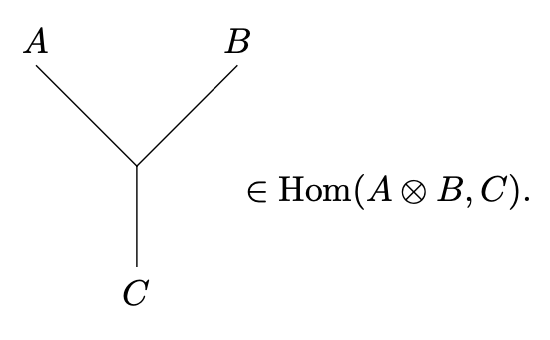This will be a non-zero morphism whenever $\dim \text{Hom}(A\otimes B,C)\geq 1$ and $0$ otherwise. Here, we are using the graphical language of string diagrams. There generators are chosen arbitrarily, except for the conditions that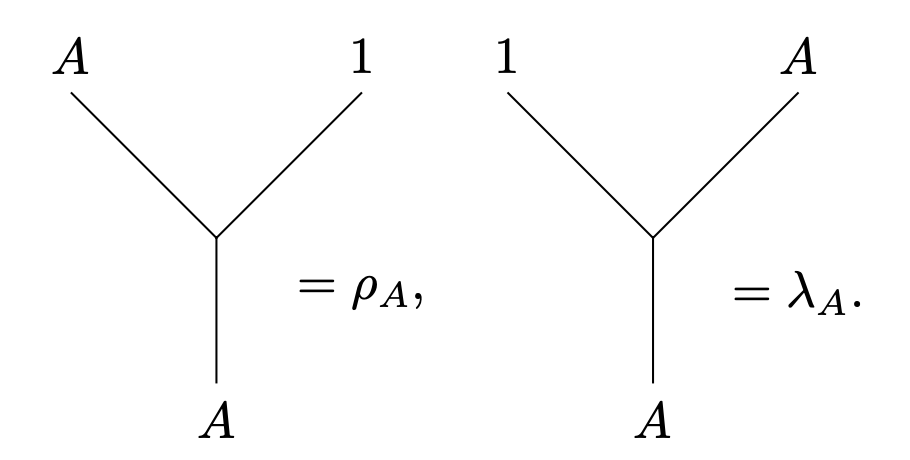where $\rho_A$ denotes the right unitor and $\lambda_A$ denotes the left unitor.

Note the key use of the fact that $\mathcal{C}$ is multiplicity free. If fusion coefficients could be $\geq 2$ then we would not have one-element bases of the spaces $\text{Hom}(A\otimes B,C)$. Instead, we would have to choose larger bases of these spaces are get matrices for our $6j$ symbols instead of complex numbers.

For all quadruples $A,B,C,D$ of simple objects, there is a basis of $\text{Hom}((A\otimes B)\otimes C,D)$ given by the symbols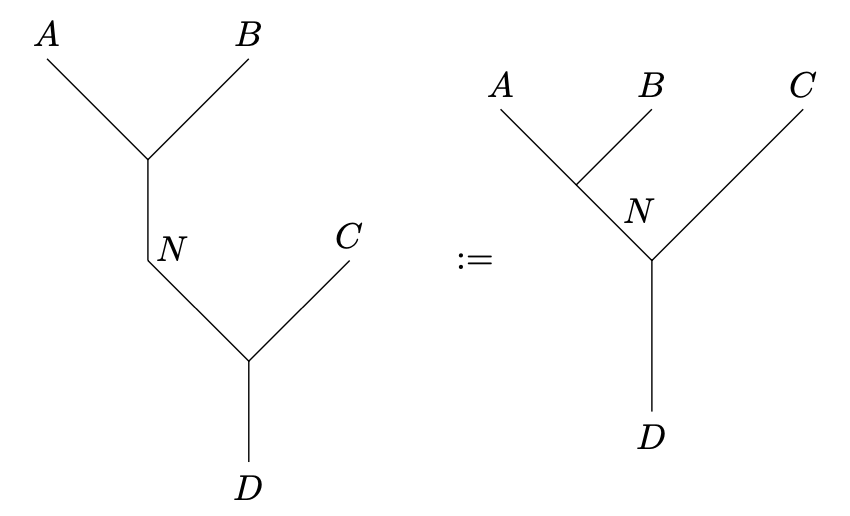and there is a basis of $\text{Hom}(A\otimes (B\otimes C),D)$.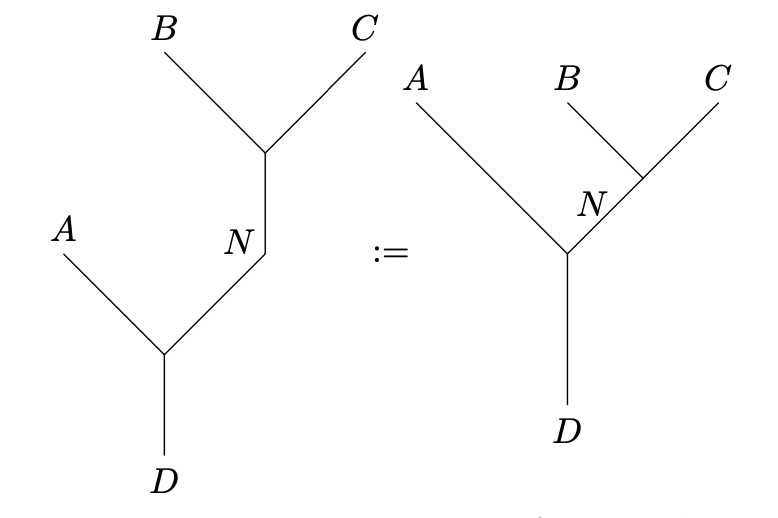where in both cases $N$ ranges over representatives of isomorphism classes of simple objects.

###### Definition

The associator $\alpha$ induces a map

$\alpha_{A,B,C}:\text{Hom}((A\otimes B)\otimes C,D)\to \text{Hom}(A\otimes (B\otimes C),D)$

for all simple objects $A,B,C,D\in\mathcal{C}$. Expressing the source and target of this map in the distinguished bases above, we can canonically identify $\alpha_{A,B,C}$ with a matrix.

The 6j symbol $F^{a,b,c}_{d;n,m}$ is defined to be the $(N,M)$th coefficient of this matrix, where $a,b,c,d,n,m$ are isomorphism classes of simple objects. That is, the $6j$ symbols are the unique complex numbers making the identity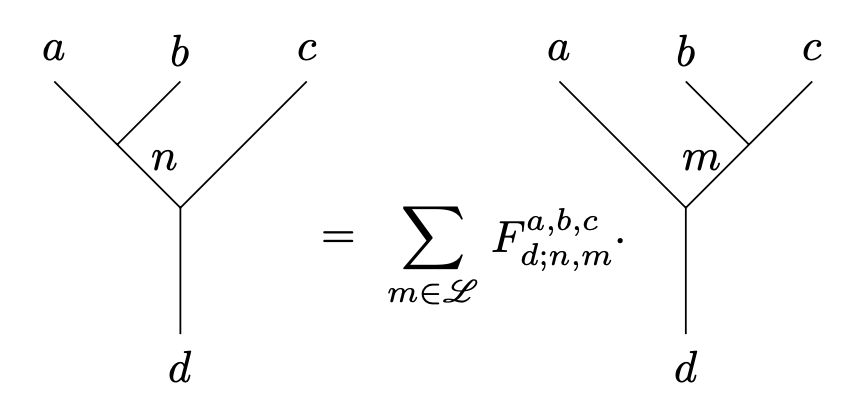holds.

## Use of Yoneda perspective

It is a key observation that one does not use the $6j$ symbols to directly encode $\alpha$. Instead, the $6j$ symbols are used to describe the action of the hom-space $\text{hom}(-,D)$.

They key point is that objects are not associated vector spaces in a linear category: hom-spaces are. Hence, when encoding maps as matrices one has to take a dual perspective from objects to morphisms.

The Yoneda lemma exactly guarantees that the data encoded is enough to recover $\alpha$. Namely, knowing the action of $\alpha$ on the hom-space $\text{hom}((A\otimes B)\otimes C,D)\to \text{hom}(A\otimes (B\otimes C),D)$ for all simple objects $D$ one gets the full data of a natural transformation $\text{hom}((A\otimes B)\otimes C,-)\to \text{hom}(A\otimes (B\otimes C),-)$ by the semisimplicity of $\mathcal{C}$. Because the Yoneda embedding is fully faithful, we can uniquely determine what map $\alpha$ must have induces this natural transformation.

Last revised on July 19, 2023 at 16:41:15. See the history of this page for a list of all contributions to it.